• 2022-03-27 22:54:12

今天主要写的函数式日期格式化函数，我们有时候调用的new Date()不是格式化的时间，可能显示不是很正常，今天这里分享一个js写的foramt（）函数，希望能对大家有所帮助！

## javascript Date format(js日期格式化)

方法一

Date.prototype.pattern=function(fmt) {
var o = {
"M+" : this.getMonth()+1, //月份
"d+" : this.getDate(), //日
"h+" : this.getHours()%12 == 0 ? 12 : this.getHours()%12, //小时
"H+" : this.getHours(), //小时
"m+" : this.getMinutes(), //分
"s+" : this.getSeconds(), //秒
"q+" : Math.floor((this.getMonth()+3)/3), //季度
"S" : this.getMilliseconds() //毫秒
};
var week = {
"0" : "/u65e5",
"1" : "/u4e00",
"2" : "/u4e8c",
"3" : "/u4e09",
"4" : "/u56db",
"5" : "/u4e94",
"6" : "/u516d"
};
if(/(y+)/.test(fmt)){
fmt=fmt.replace(RegExp.$1, (this.getFullYear()+"").substr(4 - RegExp.$1.length));
}
if(/(E+)/.test(fmt)){
fmt=fmt.replace(RegExp.$1, ((RegExp.$1.length>1) ? (RegExp.$1.length>2 ? "/u661f/u671f" : "/u5468") : "")+week[this.getDay()+""]); } for(var k in o){ if(new RegExp("("+ k +")").test(fmt)){ fmt = fmt.replace(RegExp.$1, (RegExp.$1.length==1) ? (o[k]) : (("00"+ o[k]).substr((""+ o[k]).length))); } } return fmt; }  调用方法 var date = new Date(); window.alert(date.pattern("yyyy-MM-dd hh:mm:ss")); 方法二： // 对Date的扩展，将 Date 转化为指定格式的String // 月(M)、日(d)、小时(h)、分(m)、秒(s)、季度(q) 可以用 1-2 个占位符， // 年(y)可以用 1-4 个占位符，毫秒(S)只能用 1 个占位符(是 1-3 位的数字) // 例子： // (new Date()).Format("yyyy-MM-dd hh:mm:ss.S") ==> 2006-07-02 08:09:04.423 // (new Date()).Format("yyyy-M-d h:m:s.S") ==> 2006-7-2 8:9:4.18 Date.prototype.Format = function (fmt) { var o = { "M+": this.getMonth() + 1, //月份 "d+": this.getDate(), //日 "h+": this.getHours(), //小时 "m+": this.getMinutes(), //分 "s+": this.getSeconds(), //秒 "q+": Math.floor((this.getMonth() + 3) / 3), //季度 "S": this.getMilliseconds() //毫秒 }; if (/(y+)/.test(fmt)) fmt = fmt.replace(RegExp.$1, (this.getFullYear() + "").substr(4 - RegExp.$1.length)); for (var k in o) if (new RegExp("(" + k + ")").test(fmt)) fmt = fmt.replace(RegExp.$1, (RegExp.$1.length == 1) ? (o[k]) : (("00" + o[k]).substr(("" + o[k]).length))); return fmt; } 调用方法：  var haorooms1 = new Date().Format("yyyy-MM-dd"); var haorooms2= new Date().Format("yyyy-MM-dd hh:mm:ss"); alert(haorooms1 ); alert(haorooms2); ## 小结 上面两种方法来源于网络，经过验证测试都是可以用的，也比较方便，大家在使用的时候，可以将其进行封装，在你的函数中，直接调用就可以了，这两种时间格式化函数方法还是比较好的！ 有什么更好的办法，可以留言交流，谢谢！ 更多相关内容 • 主要介绍了javascript中Date format,即js日期格式化的方法.实例总结了三种常见的JavaScript日期格式化技巧,需要的朋友可以参考下 • 在上篇文章给大家介绍了js对Date对象的操作的问题(生成一个倒数7天的数组)，本篇介绍有关js日期格式化之javascript Date format，本文通过三种方法给大家讲解，具体内容请看下文。 方法一： // 对Date的扩展，将 ... • 主要介绍的是javascript时间格式format函数，我们有时候调用的new Date()不是格式化的时间，可能显示不是很正常，那么这时候就需要格式化时间，今天这里分享一个javascript的foramt()函数，有需要的可以参考借鉴。 • JQuery 的一个日期格式化插件,支持"MM-dd HH:mm"等格式的设定;并支持日期时间转义,即距当前过去了多久,如"3分钟前","1天前","2周前". • JS 日期格式化和加减,适用于将日期格式转化为yyyy-MM-DD HH:mm:ss格式,以及日期的加减 • 主要介绍了javascript日期格式化方法,实例总结了JavaScript针对日期与时间的格式化操作技巧,具有一定参考借鉴价值,需要的朋友可以参考下 • 本文给大家汇总介绍了javascript格式日期时间的几种常用方法，个人对最后一种个性化输出时间比较有兴趣，基本上只要项目中能用到都是使用这种，推荐给小伙伴们。 • js通用日期格式化工具，直接引用该工具类就可以使用，方便快捷，建议大家收藏此工具类，该工具很好的解决了前端页面显示日期格式问题 • javascript格式日期，将日期型转为字符串，字符串转为日期型，格式自由。 • 封装一个time.js文件，包含8种格式，需要传两个参数，时间戳str以及格式化的类型type，日期补零的方法用到es6语法中的padStart（length,‘字符’）,第一个参数是指定生成的字符串的最小长度，第二个参数是用来补全的... 封装一个time.js文件，包含8种格式，需要传两个参数，时间戳str以及格式化的类型type，日期补零的方法用到es6语法中的padStart（length,‘字符’）,第一个参数是指定生成的字符串的最小长度，第二个参数是用来补全的字符串。如果没有指定第二个参数，默认用空格填充。需要注意的是需要将获取到的年月日转为字符串，可以直接拼接一个引号’’。 // type:0 =>2021-09-12 默认 // type:1 =>2021-09-12 10:34:12 // type:2 =>09-12 10:34:12 // type:3 =>09-12 // type:4 =>2021/09/12 // type:5 =>2021/09/12 10:34:12 // type:6 =>09/12 10:34:12 // type:7 =>09/12 var filterTime = function(str,type = 0) { console.log('str',str) console.log('type',type) let date = new Date(str) let y = date.getFullYear(); let m = (date.getMonth()+1 + '').padStart(2,'0'); let d = (date.getDate() + '').padStart(2,'0'); let hh = (date.getHours() + '').padStart(2,'0') let mm = (date.getMinutes() + '').padStart(2,'0') let ss = (date.getSeconds() + '').padStart(2,'0') let time; switch (type) { case 0: time = ${y}-${m}-${d};
break;
case 1:
time = ${y}-${m}-${d}${hh}:${mm}:${ss};
break;
case 2:
time = ${m}-${d} ${hh}:${mm}:${ss}; break; case 3: time = ${m}-${d}; break; case 4: time = ${y}/${m}/${d};
break;
case 5:
time = ${y}/${m}/${d}${hh}:${mm}:${ss};
break;
case 6:
time = ${m}/${d} ${hh}:${mm}:${ss}; break; case 7: time = ${m}/${d}; break; } return time; } export { filterTime }  展开全文• js虽然提供了各种获取时间Date对象的不同属性方法，如：getDate 方法 | getDay 方法 | getFullYear 方法 | getHours 方法 … … 等等，但是却没有像java那样提供一个方法来供用户来根据自身提供的模板（pattern），... • javascript提供的日期格式化太简单,一般应用都需要自己实现格式化方法.下面是我想出的一个格式化处理方案,应该是可以满足常用的需求.日期模板中可以使用任意分隔符,还可以使用文字作为分隔符,甚至支持类似yyyyMMdd之... • http://www.cnblogs.com/GuominQiu 代码如下: //————————————————————————— //判断日期格式是否正确 //返回值是错误信息, 无错误信息即表示合法日期字符串 function isDateString(strDate... • ## JS日期格式化转换方法 万次阅读 多人点赞 2018-04-21 17:31:21 1. 将日期转换为指定的格式：比如转换成 年月日时分秒 这种格式：yyyy-MM-dd hh:mm:ss 或者 yyyy-MM-dd。当然是网上的方法，只是总结下。 可以为Date原型添加如下的方法： Date.prototype.format = function(fmt)... 1. 将日期转换为指定的格式：比如转换成 年月日时分秒 这种格式：yyyy-MM-dd hh:mm:ss 或者 yyyy-MM-dd。当然是网上的方法，只是总结下。 可以为Date原型添加如下的方法： Date.prototype.format = function(fmt) { var o = { "M+" : this.getMonth()+1, //月份 "d+" : this.getDate(), //日 "h+" : this.getHours(), //小时 "m+" : this.getMinutes(), //分 "s+" : this.getSeconds(), //秒 "q+" : Math.floor((this.getMonth()+3)/3), //季度 "S" : this.getMilliseconds() //毫秒 }; if(/(y+)/.test(fmt)) { fmt=fmt.replace(RegExp.$1, (this.getFullYear()+"").substr(4 - RegExp.$1.length)); } for(var k in o) { if(new RegExp("("+ k +")").test(fmt)){ fmt = fmt.replace(RegExp.$1, (RegExp.\$1.length==1) ? (o[k]) : (("00"+ o[k]).substr((""+ o[k]).length)));
}
}
return fmt;
}       

比如我们可以这样调用下：

var time1 = new Date().format("yyyy-MM-dd hh:mm:ss");
console.log(time1);

运行如下：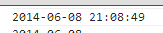也可以转换成 ”年月日”的格式

var time2 = new Date().format("yyyy-MM-dd");
console.log(time2);

运行如下：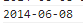2. 将指定的日期转换为"年月日"的格式，代码如下：

var oldTime = (new Date("2012/12/25 20:11:11")).getTime();
var curTime = new Date(oldTime).format("yyyy-MM-dd");
console.log(curTime);

运行如下：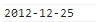3. 将 "时间戳" 转换为 "年月日" 的格式.

比如如下代码：

var da = 1402233166999;
da = new Date(da);
var year = da.getFullYear()+'年';
var month = da.getMonth()+1+'月';
var date = da.getDate()+'日';
console.log([year,month,date].join('-'));

运行如下：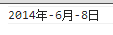展开全文date
• 日期格式化相信对于大家来说再熟悉不过，最近工作中自己利用Javascript就写了一个，现在将实现的代码分享给大家，希望对有需要的朋友们能有所帮助，感兴趣的朋友们下面来一起看看吧。
•代码
• NULL 博文链接：https://anole1982.iteye.com/blog/1855800
• 介绍了JS日期格式的验证实例，有需要的朋友可以参考一下
• 下午在搞MVC和EXTJS的日期格式互相转换遇到了问题，我们从.NET服务器端序列化一个DateTime对象的结果是一个字符串格式，如 ‘/Date(1335258540000)/’ 这样的字串。 整数1335258540000实际上是一个1970 年 1 月 1 日...
• Js日期格式化 年月日时分秒 function changeDateToStr1(date){ var y = date.getFullYear(); var m = date.getMonth() + 1; m = m < 10 ? ('0' + m) : m; var d = date.getDate(); d = d < 10 ? ('0' + ...

### Js日期格式化 年月日时分秒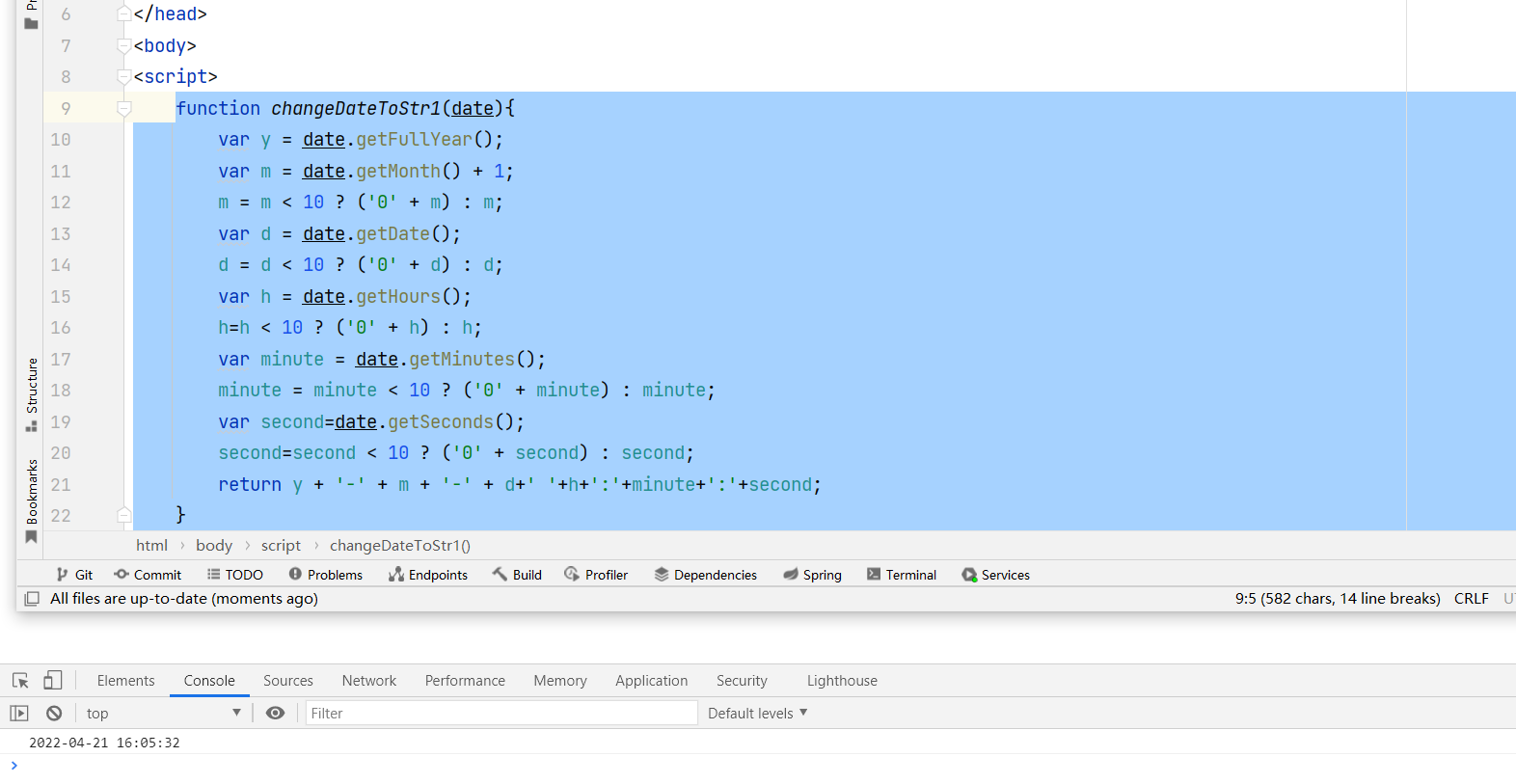function changeDateToStr1(date){
var y = date.getFullYear();
var m = date.getMonth() + 1;
m = m < 10 ? ('0' + m) : m;
var d = date.getDate();
d = d < 10 ? ('0' + d) : d;
var h = date.getHours();
h=h < 10 ? ('0' + h) : h;
var minute = date.getMinutes();
minute = minute < 10 ? ('0' + minute) : minute;
var second=date.getSeconds();
second=second < 10 ? ('0' + second) : second;
return y + '-' + m + '-' + d+' '+h+':'+minute+':'+second;
}
console.log(changeDateToStr1(new Date()))

`
展开全文• 主要介绍了vue.js将时间戳转化为日期格式的实现代码,非常不错，具有一定的参考借鉴价值，需要的朋友可以参考下
• 本文实例为大家分享了js时间戳与日期格式之间相互转换的代码，供大家参考，具体内容如下 1. 将时间戳转换成日期格式 // 简单的一句代码 var date = new Date(时间戳); //获取一个时间对象 /** 1. 下面是获取时间...
• I have a date with the format Sun May 11,2014 . 我有一个日期为Sun May 11,2014 。 How can I convert it to 20date...